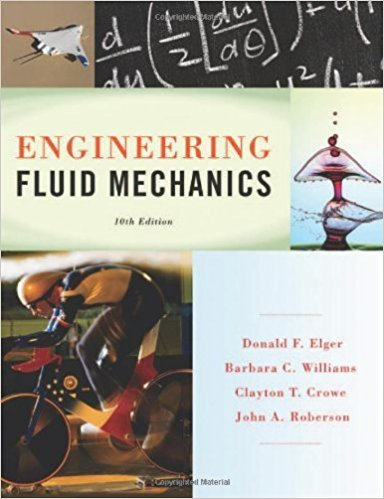×
×

# Density is (select all that apply) a. weight/volume b. mass/volume c. volume/mass dISBN: 9781118164297 288

## Solution for problem 2.3 Chapter 2

Engineering Fluid Mechanics | 10th Edition

• Textbook Solutions
• 2901 Step-by-step solutions solved by professors and subject experts
• Get 24/7 help from StudySoup virtual teaching assistantsEngineering Fluid Mechanics | 10th Edition

4 5 1 335 Reviews
16
3
Problem 2.3

Density is (select all that apply) a. weight/volume b. mass/volume c. volume/mass d. mass/weight

Step-by-Step Solution:
Step 1 of 3

Thursday, September 22, 2016 7 Ideas - Isotropic Property of Space :- Whenever an object moves in two dimensions (directions) simultaneously, motion in one dimension does not affect motion in the other. - Forces = Push or Pull :- 4 Forces of Nature Gravity Electromagnetic Strong Interacting (Nuclear) Weak Interacting (Nuclear) Gravity :- -Weakest...

Step 2 of 3

Step 3 of 3

##### ISBN: 9781118164297

This textbook survival guide was created for the textbook: Engineering Fluid Mechanics, edition: 10. The answer to “Density is (select all that apply) a. weight/volume b. mass/volume c. volume/mass d. mass/weight” is broken down into a number of easy to follow steps, and 14 words. The full step-by-step solution to problem: 2.3 from chapter: 2 was answered by , our top Physics solution expert on 01/25/18, 04:53PM. This full solution covers the following key subjects: . This expansive textbook survival guide covers 16 chapters, and 1172 solutions. Engineering Fluid Mechanics was written by and is associated to the ISBN: 9781118164297. Since the solution to 2.3 from 2 chapter was answered, more than 230 students have viewed the full step-by-step answer.

Unlock Textbook Solution